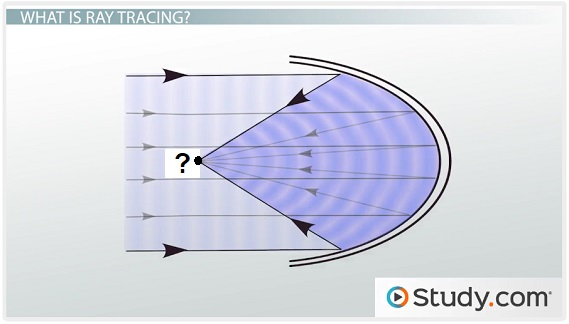# Basics of Optics Flashcards

Basics of Optics Flashcards
1/17 (missed) 0 0
Create Your Account To Continue Studying

As a member, you'll also get unlimited access to over 79,000 lessons in math, English, science, history, and more. Plus, get practice tests, quizzes, and personalized coaching to help you succeed.

Try it risk-free for 30 days. Cancel anytime
Convex Lens

A type of lens that bends light in such a way that the light rays converge to a point

Focal length is a positive value

Got it
An object is put 2 m in front of a concave mirror with a focal point of 6 m. Determine how much smaller or bigger the reflected object is.

1/do + 1/f = 1/di

1/-2 + 1/6 = 1/di

1/(1/-2 + 1/6) = di

di = -3

M = di/do = -3/-2 = 1.5 times bigger

Got it
A 10 m image is put in front of a concave mirror with a focal point of 5 m. Determine where the reflected image is in relation to the mirror.

1/do + 1/f = 1/di

1/-2 + 1/6 = 1/di

1/(1/-2 + 1/6) = di

di = -3 = 3 m behind the mirror

Got it
Virtual Image (Mirrors)

An image made by a mirror that cannot be projected onto a screen because it forms behind the mirror

Got it
Real Image (Mirrors)

An image made by a mirror that can be projected onto a screen because it forms in front of the mirror

Got it
Law of Reflection

States that the angle at which a light ray hits a surface (incident angle) and the angle at which it bounces away from the surface (reflected angle) are equal

Basis of ray tracing

Got it
Concave Mirror

A mirror that takes the shape of the inside of a spherical curved surface

Produces different images depending on where the object is in relation to the mirror's focal point

Got it
Convex Mirror

A mirror that takes the shape of the outside of a spherical curved surface

Produces image that are upright, virtual (behind the mirror), and smaller than the object

Got it

or choose a specific lesson: See all lessons in this chapter
17 cards in set

## Flashcard Content Overview

Have you ever wondered how a funhouse mirror worked? Or how the lenses in a microscope magnify images? The flashcards in this set review the basic of optics, which includes how mirrors and lenses work. The will also help you review how polarization and thin film interference works.

Front
Back
Convex Mirror

A mirror that takes the shape of the outside of a spherical curved surface

Produces image that are upright, virtual (behind the mirror), and smaller than the object

Concave Mirror

A mirror that takes the shape of the inside of a spherical curved surface

Produces different images depending on where the object is in relation to the mirror's focal point

Law of Reflection

States that the angle at which a light ray hits a surface (incident angle) and the angle at which it bounces away from the surface (reflected angle) are equal

Basis of ray tracing

Real Image (Mirrors)

An image made by a mirror that can be projected onto a screen because it forms in front of the mirror

Virtual Image (Mirrors)

An image made by a mirror that cannot be projected onto a screen because it forms behind the mirror

A 10 m image is put in front of a concave mirror with a focal point of 5 m. Determine where the reflected image is in relation to the mirror.

1/do + 1/f = 1/di

1/-2 + 1/6 = 1/di

1/(1/-2 + 1/6) = di

di = -3 = 3 m behind the mirror

An object is put 2 m in front of a concave mirror with a focal point of 6 m. Determine how much smaller or bigger the reflected object is.

1/do + 1/f = 1/di

1/-2 + 1/6 = 1/di

1/(1/-2 + 1/6) = di

di = -3

M = di/do = -3/-2 = 1.5 times bigger

Convex Lens

A type of lens that bends light in such a way that the light rays converge to a point

Focal length is a positive value

Concave Lens

A type of lens that bends light in such a way that the light rays diverge

Focal length is a negative value

Virtual Image (Lenses)

An image made by a lens that cannot be projected onto a screen because it forms on the same side of the lens as the object

Represented by a negative image distance

Thin Lens Equation

1/do + 1/di = 1/f

An object is placed 5 cm above an object and becomes clear when looked at from a distance of 15 cm above the glass. Determine the focal length of the magnifying glass.

1/do + 1/di = 1/f

1/5 + 1/15 = 1/f

1/(1/5 + 1/15) = f

f = 3.75 cm

An object is placed 5 cm above an object and becomes clear when looked at from a distance of 15 cm above the glass. Determine the magnification of the magnifying glass.

M = -di/do = -15/5 = -3 = 3

Polarizer/Polarizing Filter

Found in places like sunglasses and camera lens filters

Blocks light that oscillates in one direction but not another, based on orientation and angle of slits in the polarizer

A diamond has an index of refraction of 2.42. Determine the angle at which light becomes polarized when it is shined onto a diamond through air.

n1 = air = 1.0

n2 = diamond = 2.42

tanθB = 2.42/1.0

θB = tan-1 (2.42/1.0) = 67.5°

Path Difference

Occurs in thin film interference when beams of light arrive at the boundary between different materials sandwiched together and some light passes through while some reflects

Label the diagram.Light rays that hit a concave mirror parallel to the principal axis converge at the focal point.

To unlock this flashcard set you must be a Study.com Member.